# JEE Main Circle Previous Year Questions With Solutions

Circle is the curve traced out by a point that moves in a plane so that its distance from a given point is always constant. The general equation of a circle with centre (–g, –f) and radius √(g2+f2-c) is x2 + y2 + 2gx + 2fy + c = 0. Students can check few more relevant concepts below. About 1-2 questions asked in the JEE exam from this topic. JEE previous year solved questions on Circle can be accessed by students to begin with their preparation for JEE entrance. This set of questions is formulated in an easy and understandable way, which help students in solving problems effortlessly. JEE chapter-wise solutions are always a best study material, which can be used by aspirants for quick revision before the exam to check their preparation level. Solving previous year question papers boost confidence among aspirants, so, they are advised to practice JEE Previous year chapter-wise question papers to strengthen their skills.

### Important Points to Remember:

 1. The equation of a circle with its center at C(x0, y0) and radius r is: (x – x0)2 + (y – y0)2 = r2 2. For general circle, the equation of the chord is x1x + y1y + g(x1 + x) + f(y1 + y) + c = 0 3. Equation of the circle with points P(x1, y1) and Q(x2, y2) as extremities of a diameter is (x – x1) (x – x2) + (y – y1)(y – y2) = 0. 4. Chords are equidistant from the center of a circle iff they are equal in length. 5. Angle between the tangent and the radius is 90°. 6. Angles in the same segment are equal. 7. If diameter or radius of a circle is perpendicular to it’s chord, then it divides the chord into two equal parts. The converse is also true. 8. The arcs intercepted by two congruent chords in a circle are congruent.

## JEE Main Past Year Questions With Solutions on Circle

Question 1: Let the tangents drawn from the origin to the circle x2 + y2 – 8x – 4y + 16 = 0 touch it at the point A and B. The (AB)2 is equal to

(a) 32/5                   (b) 64/5                     (c) 52/5                      (d) 56/5

Solution:

x2 + y2 – 8x – 4y + 16 = 0

Rearranging above equation, we get

(x – 4)2+ (y – 2)2 = 4

Centre = (4, 2) and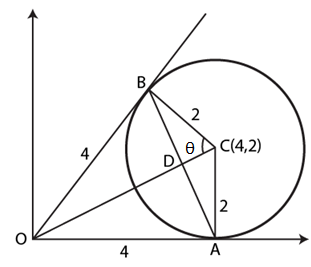OA = OB = 4

In triangle, OBC,

tan θ = 4/2 = 2

and sinθ = 2/√5

In triangle, BDC

sinθ = BD/2 => BD = 4/√5

Length of chord of contact = AB = 8/√5

Question 2: The circle passing through (1, -2) and touching the axis of x at (3, 0) also passes through the point:

(a) (–5, 2)            (b) (2, –5)                    (c) (5, –2)                 (d) (–2, 5)

Solution: The equation of circle passing through the point (a,b) and having radius r is (x – a)2 + (y – b)2 = r2

Since given circle touches the x-axis at (3, 0) and passes through the point (1, -2).

Find radius of the circle using distance formula,

So, (1 – 3) 2 + (r + 2) 2 = r2

4 + r2 + 4 + 4r – r2 = 0

=> r = 2

So, circle is (x -3)2 + (y + 2)2 = 4

Point (5, -2) satisfy the equation.

Question 3: Let C be the circle with centre at (1, 1) and radius = 1. If T is the circle centred at (0, y), passing through origin and touching the circle C externally, then the radius of T is equal to :

(a) $\frac{\sqrt{3}}{\sqrt{2}}$ (b)$\frac{\sqrt{3}}{2}$ (c) $\frac{1}{2}$ (d) $\frac{1}{4}$

Solution:

C be the circle with centre at (1, 1) and radius = 1.

T is the circle centred at (0, y), passing through origin and touching the circle C externally.

Let r1 and r2 be the radius of two circles.

CT = r1 + r2 = √[(1+(1-y)2] = 1 + y

Where “y” be the radius of circle T.

Squaring both sides, we have

1 + 1 + y2 – 2y = 1 + y2 + 2y

=> y = 1/4

Question 4: The centres of those circles which touch the circle, x2 + y2 – 8x – 8y – 4 = 0, externally and also touch the x-axis, lie on,

(a) A parabola

(b) An ellipse which is not a circle

(c) A circle

(d) A hyperbola

Solution:

The general equation of the circle with center (h, k) and radius r is, (x – h)2 + (y – k)2 = r2 …(1)

r1 = √[g2+f2-c] = √[16+16+4] = √36 = 6

Where r1 is the radius of given circle.

Since circle (1) touch the given circle.

(h – 4)2 + (k – 4)2 = (6 + k)2

Solving above equation, we have

(h – 4)2 = 20k + 20

Replace (h, k) by (x, y)

(x – 4)2 = 4(5y + 5)

Which is locus of parabola.

Question 5: If a circle “C” passing through any point (4, 0) touches the circle x2 + y2 + 4x – 6y – 12 = 0 externally at a point (1,-1), then what will be the radius of the circle.

Solution:

We know, equation of family of circles: (x – 1)2 + (y + 1)2 + λ(x2 + y2 + 4x – 6y – 12) = 0 …(1)

(4, 0) satisfy the equation as it passes through it.

=>9 + 1 + λ(16 +16 – 12) = 0

=> λ = -1/2

Therefore, (1)=> x2 + y2 – 2x + 2y + 2 – 1/2 (x2 + y2 + 4x – 6y – 12) = 0

=> x2 + y2 – 8x + 10y + 16 = 0

Now, Radius, r = √[16+25-16] = √25 = 5

Question 6: A circle touches the y-axis at the point (0, 4) and passes through point (2,0). which of the following lines is not a tangent to the circle?

(a) 4x – 3y + 17 = 0

(b) 3x + 4y – 6 = 0

(c) 4x + 3y – 8 = 0

(d) 3x – 4y – 24 = 0

Solution: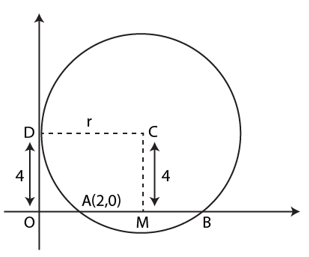From figure,

OD2 = OA x OB

=> 16 = 2 x OB

=> OB = 8

So, AB = 6

AM = 3 and CM = 4

=> CA = 5

Therefore, OM = 5

Now, we have center = (5, 4) and radius = 5

Check for all the given options.

4x + 3y – 8 = 0 is not a tangent.

so, [20+12-8]/√[32+42] = 24/5

Question 7: The number of common tangents to the circle x2 + y2 – 4x – 6y – 12 = 0 and x2 + y2 + 6x + 18y + 26 = 0, is :

(a) 3                        (b) 4                         (c) 1                        (d) 2

Solution: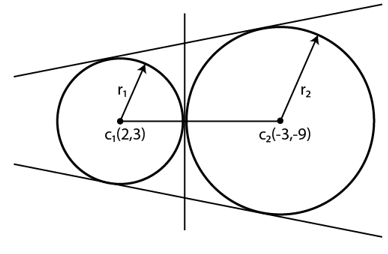From diagram,

C1C2 = 13

(Using distance formula)

Now, r1 = √[4+9+12] = 5 and r2 = √[9+81-26] = 8

C1 C2 = r1 + r2 is case of external touching. So, number of common tangents can be drawn = 3.

Question 8: Three circles of radii, a, b, c (a<b<c) touch each other externally and have x−axis as a common tangent then

(a) a, b, c are in A.P.

(b) 1/√b = 1/√a + 1/√c

(c) √a, √b, √c are in A.P.

(d) 1/√a = 1/√b + 1/√c

Solution: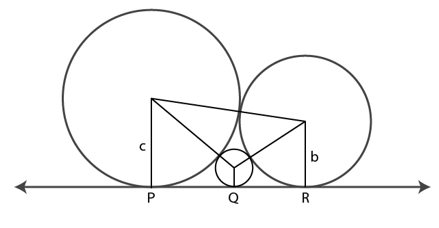From figure,

PQ + QR = PR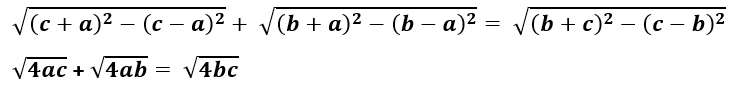Dividing each side with √(4abc)

=> 1/√b + 1/√c = 1/√a

Question 9: If the circle x2 + y2 – 6x – 8y + (25 – a2) = 0 touches the axis of x, then “a” equals : –

(a) ± 4                             (b) ±3                   (c) 0                 (d) ±2

Solution:

Circle x2 + y2 – 6x – 8y + (25 – a2) = 0 touches the axis of x.

On rearranging given equation, we have

(x2 – 6x + 9) + (y2 – 8y + 16) = a2

=>(x – 3)2 + (y – 4)2 = a2 …(1)

We know the general equation of circle passing through (a, b) having radius “r” is: (x – a)2 + (y – b)2 = r2

Comparing with (1), we get

a = 3, b = 4 and r = ±a

Since, circle touches the axis of x, then r = b = 4

Therefore, Radius = r = ± 4

Question 10: If the curves x2 – 6x + y2 + 8 = 0 and x2 − 8y + y2 + 16 − k = 0,(k > 0) touch each other at a point, then the largest value of k is _________.

Solution: Two circles touch each other if C1C2 = |r1 ± r2|

√k + 1 = 5 or |√k − 1| = 5

This implies, k = 16 or 36

Maximum value of k is 36

Question 11: Let the orthocentre and centroid of a triangle be A(-3, 5) and B(3, 3) respectively. If C is the circumcentre of this triangle, then the radius of the circle having line segment AC as diameter, is:

(a) 2√10                              (b) 3√(5/2)                         (c) 3√5/2                    (d) √10

Solution: Let C be any point having coordinates (h, k).

Using section formula, we have

[2h+(-3)]/3 = 3, [2k+5]/3 = 3

=> h = 6 and k = 2

So, point C is (6, 2).

Here, Point A is (-3, 5)

=> Diameter = AC = √(92+32) = 3√10

Therefore, radius = diameter/2 = 3√10/2 = 3√(5/2) units

Question 12: If the tangent at (1, 7) to the curve x2 = y – 6 touches the circle x2 + y2 + 16x + 12y + c = 0 then the value of c is

(a) 79                 (b) 83                    (c) 95                    (d) 105

Solution:

Given equation is x2 = y – 6

Or y = x2 + 6

Find slope of tangent at (1, 7)

=>Let m = dy/dx = 2x

=>m = 2x 1 = 2

Equation of tangent at the point (1,7) is

(y – 7) = 2(x – 1)

=>y – 7 = 2x – 2

=> 2x – y + 5 = 0

Given equation of circle can be rearranged as:

x2 + y2 + 16x + 12y + c = 0 => (x + 8)2 + (y + 6)2 + c – 64 – 36 = 0

=>(x + 8) 2 + (y + 6) 2 = 100 – c

If tangent touches the circle, then radius is :

Distance of (-8, -6) from 2x – y + 6 = 0

So, d = $|\frac{2(-8)-(-6)}{\sqrt{4+1}}|=|\sqrt{5}|$

Radius = √(100-c) = √5 => c = 95